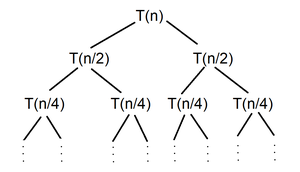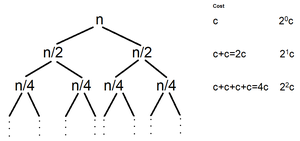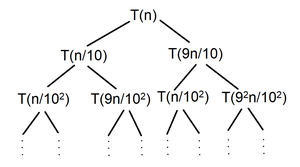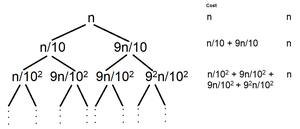# How to solve time complexity Recurrence Relations using Recursion Tree method?

• Difficulty Level : Medium
• Last Updated : 24 Nov, 2021

The Recursion Tree Method is a way of solving recurrence relations. In this method, a recurrence relation is converted into recursive trees. Each node represents the cost incurred at various levels of recursion. To find the total cost, costs of all levels are summed up.

Steps to solve recurrence relation using recursion tree method:

1. Draw a recursive tree for given recurrence relation
2. Calculate the cost at each level and count the total no of levels in the recursion tree.
3. Count the total number of nodes in the last level and calculate the cost of the last level
4. Sum up the cost of all the levels in the recursive tree

Let us see how to solve these recurrence relations with the help of some examples:

Question 1: T(n) = 2T(n/2) + c

Solution:

• Step 1: Draw a recursive treeRecursion Tree

• Step 2: Calculate the work done or cost at each level and count total no of levels in recursion treeRecursive Tree with each level cost

Count the total number of levels –

Choose the longest path from root node to leaf node

n/20 -→ n/21 -→ n/22 -→ ……… -→ n/2k

Size of problem at last level = n/2k

At last level size of problem becomes 1

n/2k = 1

2k = n

k = log2(n)

Total no of levels  in recursive tree = k +1 = log2(n) + 1

• Step 3: Count total number of nodes in the last level and calculate cost of last level

No. of nodes at level 0 = 20 = 1

No. of nodes at level 1 = 21 = 2

………………………………………………………

No. of nodes at level log2(n) = 2log2(n) = nlog2(2) = n

Cost of sub problems at level log2(n) (last level) = nxT(1) = nx1 = n

• Step 4: Sum up the cost all the levels in recursive tree

T(n) = c + 2c + 4c + —- + (no. of levels-1) times + last level cost

= c + 2c + 4c + —- + log2(n) times + Θ(n)

= c(1 + 2 + 4 + —- + log2(n) times) + Θ(n)

1 + 2 + 4 + —– + log2(n) times –> 20 + 21 + 22 + —– + log2(n) times –> Geometric Progression(G.P.)

= c(n) + Θ(n)

Thus, T(n) = Θ(n)

Question 2: T(n) = T(n/10) + T(9n/10) + n

Solution:

• Step 1: Draw a recursive treeRecursive Tree

• Step 2: Calculate the work done or cost at each level and count total no of levels in recursion treeRecursion Tree with each level cost

Count the total number of levels –

Choose the longest path from root node to leaf node

(9/10)0n –> (9/10)1n –> (9/10)2n –> ……… –> (9/10)kn

Size of problem at last level = (9/10)kn

At last level size of problem becomes 1

(9/10)kn = 1

(9/10)k = 1/n

k = log10/9(n)

Total no of levels in recursive tree = k +1 = log10/9(n) + 1

• Step 3: Count total number of nodes in the last level and calculate cost of last level

No. of nodes at level 0 = 20 = 1

No. of nodes at level 1 = 21 = 2

………………………………………………………

No. of nodes at level log10/9(n) = 2log10/9(n) = nlog10/9(2)

Cost of sub problems at level log2(n) (last level) = nlog10/9(2) x T(1) = nlog10/9(2) x 1 = nlog10/9(2)

• Step 4: Sum up the cost all the levels in recursive tree

T(n) = n + n + n + —- + (no. of levels – 1) times + last level cost

= n + n + n + —- + log10/9(n) times + Θ(nlog10/9(2))

= nlog10/9(n) + Θ(nlog10/9(2))

Thus, T(n) = Θ(nlog10/9(n))

My Personal Notes arrow_drop_up